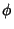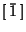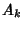Noble Number

A noble number is defined as an Irrational Number which has a Continued Fraction which becomes an infinite sequence of 1s at some point,The prototype is the Golden Ratiowhose Continued Fraction is composed entirely of 1s,. Any noble number can written aswhereandare the Numerator and Denominator of theth Convergent of. The noble numbers are a Subfield of.

Schroeder, M. Noble and Near Noble Numbers.'' In Fractals, Chaos, Power Laws: Minutes from an Infinite Paradise. New York: W. H. Freeman, pp. 392-394, 1991.